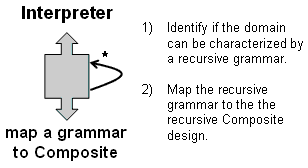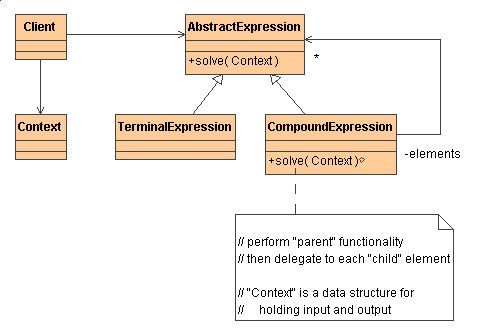# Interpreter

### Intent

• Given a language, define a representation for its grammar along with an interpreter that uses the representation to interpret sentences in the language. [GoF, 243]
• Map a domain to a language, the language to a grammar, and the grammar to a hierarchical object-oriented design.

### Problem

A class of problems occurs repeatedly in a well-defined and well-understood domain. If the domain were characterized with a "language", then problems could be easily solved with an interpretation "engine".

### Structure SummaryStructure category:
recursive composition
Similar patterns:   Composite   Decorator   Chain of Responsibility

### Discussion

The Interpreter pattern discusses: defining a domain language (i.e. problem characterization) as a simple language grammar, representing domain rules as language sentences, and interpreting these sentences to solve the problem. The pattern uses a class to represent each grammar rule. And since grammars are usually hierarchical in structure, an inheritance hierarchy of rule classes maps nicely.

An abstract base class specifies the method interpret(). Each concrete subclass implements interpret() by accepting (as an argument) the current state of the language stream, and adding its contribution to the problem solving process.

### StructureInterpreter suggests modeling the domain with a recursive grammar. Each rule in the grammar is either a “composite” (a rule that references other rules) or a terminal (a leaf node in a tree structure). Interpreter relies on the recursive traversal of the Composite pattern to interpret the “sentences” it is asked to process.### Example

The Intepreter pattern defines a grammatical representation for a language and an interpreter to interpret the grammar. Musicians are examples of Interpreters. The pitch of a sound and its duration can be represented in musical notation on a staff. This notation provides the language of music. Musicians playing the music from the score are able to reproduce the original pitch and duration of each sound represented. [Michael Duell, "Non-software examples of software design patterns", Object Magazine, Jul 97, p54]### Check list

1. Decide if a "little language" offers a justifiable return on investment.
2. Define a grammar for the language.
3. Map each production in the grammar to a class.
4. Organize the suite of classes into the structure of the Composite pattern.
5. Define an `interpret(Context)` method in the Composite hierarchy.
6. The `Context` object encapsulates the current state of the input and output as the former is parsed and the latter is accumulated. It is manipulated by each grammar class as the "interpreting" process transforms the input into the output.

### Before and after

BeforeAfter
```// This is an adaptation of a design that appeared in a Pascal
// data structures book.  The intent was to use stacks to convert
// normal "infix" syntax into "postfix" notation with operator

public class InterpreterDemo {
public static boolean precedence( char a, char b ) {
String high = "*/", low = "+-";
if (a == '(') return false;
if (a == ')'  &&  b == '(') {
System.out.println( ")-(" );
return false;
}
if (b == '(') return false;
if (b == ')') return true;
if (high.indexOf( a ) > -1  &&  low.indexOf( b )  > -1)
return true;
if (high.indexOf( a ) > -1  &&  high.indexOf( b ) > -1)
return true;
if (low.indexOf( a ) > -1   &&  low.indexOf( b )  > -1)
return true;
return false;
}
public static String convert_to_postfix( String expr ) {
Stack<Character>  op_stack  = new Stack<Character>();
StringBuffer out    = new StringBuffer();
String       opers  = "+-*/()";
char         top_sym = '+';
boolean      empty;
String[]     tokens = expr.split( " " );

for (int i = 0; i < tokens.length; i++)
if (opers.indexOf( tokens[i].charAt(0) ) == -1) {
out.append( tokens[i] );
out.append( ' ' );
} else {
while ( ! (empty = op_stack.isEmpty())
&&  precedence( top_sym = op_stack.pop(),
tokens[i].charAt(0))) {
out.append( top_sym );
out.append( ' ' );
}
if ( ! empty)
op_stack.push( top_sym );
if (empty || tokens[i].charAt(0) != ')')
op_stack.push( tokens[i].charAt(0) );
else
top_sym = op_stack.pop();
}
while ( ! op_stack.isEmpty()) {
out.append( op_stack.pop() );
out.append( ' ' );
}
return out.toString();
}
public static double process_postfix( String postfix,
HashMap<String,Integer> map ) {
Stack<Double> stack = new Stack<Double>();
String   opers  = "+-*/";
String[] tokens = postfix.split( " " );
for (int i=0; i < tokens.length; i++)
// If token is a number or variable
if (opers.indexOf( tokens[i].charAt(0) ) == -1) {
double term = 0.;
try {
term = Double.parseDouble( tokens[i] );
} catch (NumberFormatException ex) {
term = map.get( tokens[i] );
}
stack.push( term );

// If token is an operator
} else {
double b = stack.pop(), a = stack.pop();
if      (tokens[i].charAt(0) == '+') a = a + b;
else if (tokens[i].charAt(0) == '-') a = a - b;
else if (tokens[i].charAt(0) == '*') a = a * b;
else if (tokens[i].charAt(0) == '/') a = a / b;
stack.push( a );
}
return stack.pop();
}

public static void main( String[] args ) {
String infix = "C * 9 / 5 + 32";
String postfix = convert_to_postfix( infix );
System.out.println( "Infix:   " + infix );
System.out.println( "Postfix: " + postfix );
HashMap<String,Integer> map =
new HashMap<String,Integer>();
for (int i=0; i <= 100; i += 10) {
map.put( "C", i );
System.out.println( "C is " + i + ",  F is "
+ process_postfix( postfix, map ) );
}  }  }

// Infix:   C * 9 / 5 + 32
// Postfix: C 9 * 5 / 32 +
// C is 0,  F is 32.0
// C is 10,  F is 50.0
// C is 20,  F is 68.0
// C is 30,  F is 86.0
// C is 40,  F is 104.0
// C is 50,  F is 122.0
// C is 60,  F is 140.0
// C is 70,  F is 158.0
// C is 80,  F is 176.0
// C is 90,  F is 194.0
// C is 100,  F is 212.0
```

```// This is a refactoring that follows the intent of the Inter-
// preter design pattern.  All classes in the Operand hierarchy:
// implement the evaluate(context), digest some piece of the
// context argument, and return their contribution to the recur-
// sive traversal.  Applying the Interpreter pattern in this
// domain is probably inappropriate.

interface Operand {
double evaluate( HashMap<String,Integer> context );
void traverse( int level );
}

class Expression implements Operand {
private char m_operator;
public Operand left, rite;
public Expression( char op ) { m_operator = op; }
public void traverse( int level ) {
left.traverse( level+1 );
System.out.print( "" + level + m_operator + level + " " );
rite.traverse( level+1 );
}
public double evaluate( HashMap<String,Integer> context ) {
double result = 0.;
double a = left.evaluate( context );
double b = rite.evaluate( context );
if      (m_operator == '+') result = a + b;
else if (m_operator == '-') result = a - b;
else if (m_operator == '*') result = a * b;
else if (m_operator == '/') result = a / b;
return result;
}  }

class Variable implements Operand {
private String m_name;
public Variable( String name )  { m_name  = name;  }
public void traverse( int level ) {
System.out.print( m_name + " " );
}
public double evaluate( HashMap<String,Integer> context ) {
return context.get( m_name );
}  }

class Number implements Operand {
private double m_value;
public Number( double value ) { m_value = value; }
public void traverse( int level ) {
System.out.print( m_value + " " );
}
public double evaluate( HashMap context ) {
return m_value;
}  }

public class InterpreterDemo {
public static boolean precedence( char a, char b ) {
String high = "*/", low = "+-";
if (a == '(') return false;
if (a == ')'  &&  b == '(') {
System.out.println( ")-(" );
return false;
}
if (b == '(') return false;
if (b == ')') return true;
if (high.indexOf( a ) > -1  &&  low.indexOf( b )  > -1)
return true;
if (high.indexOf( a ) > -1  &&  high.indexOf( b ) > -1)
return true;
if (low.indexOf( a ) > -1   &&  low.indexOf( b )  > -1)
return true;
return false;
}
public static String convert_to_postfix( String expr ) {
Stack<Character>  op_stack  = new Stack<Character>();
StringBuffer out    = new StringBuffer();
String       opers  = "+-*/()";
char         top_sym = '+';
boolean      empty;
String[]     tokens = expr.split( " " );

for (int i = 0; i < tokens.length; i++)
if (opers.indexOf( tokens[i].charAt(0) ) == -1) {
out.append( tokens[i] );
out.append( ' ' );
} else {
while ( ! (empty = op_stack.isEmpty())
&&  precedence( top_sym = op_stack.pop(),
tokens[i].charAt(0))) {
out.append( top_sym );
out.append( ' ' );
}
if ( ! empty)
op_stack.push( top_sym );
if (empty || tokens[i].charAt(0) != ')')
op_stack.push( tokens[i].charAt(0) );
else
top_sym = op_stack.pop();
}
while ( ! op_stack.isEmpty()) {
out.append( op_stack.pop() );
out.append( ' ' );
}
return out.toString();
}
public static Operand build_syntax_tree( String tree ) {
Stack<Operand> stack = new Stack<Operand>();
String   opers  = "+-*/";
String[] tokens = tree.split( " " );
for (int i=0; i < tokens.length; i++)
// If token is a number or variable
if (opers.indexOf( tokens[i].charAt(0) ) == -1) {
Operand term = null;
try {
term = new Number( Double.parseDouble( tokens[i] ) );
} catch (NumberFormatException ex) {
term = new Variable( tokens[i] );
}
stack.push( term );

// If token is an operator
} else {
Expression expr = new Expression( tokens[i].charAt(0) );
expr.rite = stack.pop();  expr.left = stack.pop();
stack.push( expr );
}
return stack.pop();
}
public static void main( String[] args ) {
System.out.println( "celsi * 9 / 5 + thirty" );
String postfix = convert_to_postfix( "celsi * 9 / 5 + thirty" );
System.out.println( postfix );
Operand expr = build_syntax_tree( postfix );
expr.traverse( 1 );  System.out.println();
HashMap<String,Integer> map = new HashMap<String,Integer>();
map.put( "thirty", 30 );
for (int i=0; i <= 100; i += 10) {
map.put( "celsi", i );
System.out.println( "C is " + i + ",  F is "
+ expr.evaluate( map ) );
}  }  }

// celsi * 9 / 5 + thirty
// celsi 9 * 5 / thirty +
// celsi 3*3 9.0 2/2 5.0 1+1 thirty
// C is 0,  F is 30.0
// C is 10,  F is 48.0
// C is 20,  F is 66.0
// C is 30,  F is 84.0
// C is 40,  F is 102.0
// C is 50,  F is 120.0
// C is 60,  F is 138.0
// C is 70,  F is 156.0
// C is 80,  F is 174.0
// C is 90,  F is 192.0
// C is 100,  F is 210.0
```

### Rules of thumb

Considered in its most general form (i.e. an operation distributed over a class hierarchy based on the Composite pattern), nearly every use of the Composite pattern will also contain the Interpreter pattern. But the Interpreter pattern should be reserved for those cases in which you want to think of this class hierarchy as defining a language. [GoF, p255]

Interpreter can use State to define parsing contexts. [GoF, p349]

The abstract syntax tree of Interpreter is a Composite (therefore Iterator and Visitor are also applicable). [GoF, p255]

Terminal symbols within Interpreter's abstract syntax tree can be shared with Flyweight. [GoF, p255]

The pattern doesn't address parsing. When the grammar is very complex, other techniques (such as a parser) are more appropriate. [GoF, p247]